## Tamilnadu Samacheer Kalvi 10th Maths Solutions Chapter 2 Numbers and Sequences Ex 2.5

10th Maths Exercise 2.5 Samacheer Kalvi Question 1.
Check whether the following sequences are in A.P.
(i) a – 3, a – 5, a – 7, ………
(ii) $$\frac { 1 }{ 2 }$$,$$\frac { 1 }{ 3 }$$,$$\frac { 1 }{ 4 }$$,$$\frac { 1 }{ 4 }$$, ………..
(iii) 9, 13, 17, 21, 25, ………
(iv) $$\frac { -1 }{ 3 }$$,0,$$\frac { 1 }{ 3 }$$,$$\frac { 2 }{ 3 }$$,………..
(v) 1,-1, 1,-1, 1,-1,…
Solution:
To prove it is an A.P, we have to show d = t2 – t1 = t3 – t2.
(i) a – 3, a – 5, a – 7………
t1,t2,t3
d = t2 – t1 = a – 5 – (a – 3) = a – 5 – a + 3 = -2
∴ d = -2    ∴ It is an A.P.
d = t3 – t2 = a – 7 – (a – 5) = a – 7 – a + 5 = -2

(ii) $$\frac { 1 }{ 2 }$$,$$\frac { 1 }{ 3 }$$,$$\frac { 1 }{ 4 }$$,$$\frac { 1 }{ 4 }$$, ………..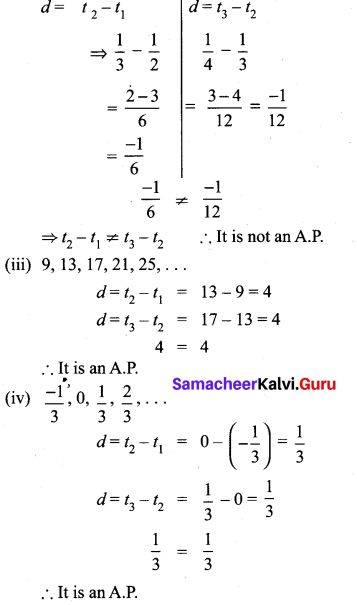(v) 1,-1, 1,-1, 1,-1,…
d = t2 – t1 = -1 -1 = -2
d = t3 – t2 = 1 – (-1) = 2
-2 ≠ 2 ∴ It is not an A.P.

Ex 2.5 Class 10 Samacheer Question 2.
First term a and common difference d are given below. Find the corresponding A.P.
(i) a = 5, d = 6
(ii) a = 7, d = 5
(iii) a = $$\frac { 3 }{ 4 }$$, d = $$\frac { 1 }{ 2 }$$
Solution:
(i) a = 5, d = 6
A.P a, a + d, a + 2d, ………
= 5, 5 + 6, 5 + 2 × 6, ………
= 5, 11, 17,…
(ii) a = 7,d = -5
A.P. = a,a + d,a + 2d,…
= 7,7 + (-5), 7 + 2(-5), ……….
= 7, 2, -3, …….,…
(iii) a = $$\frac { 3 }{ 4 }$$, d = $$\frac { 1 }{ 2 }$$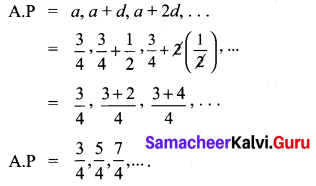Exercise 2.5 Class 10 Maths Solution Question 3.
Find the first term and common difference of the Arithmetic Progressions whose nthterms are given below

(i) tn = -3 + 2n
tn = -3 + 2 n
t1 = -3 + 2(1) = -3 + 2
= -1
t2 = -3 + 2(2) = -3 + 4
= 1
First term (a) = -1 and
Common difference
(d) = 1 – (-1) = 1 + 1 = 2

(ii) tn = 4 – 7n
tn = 4 – 7n
t1 = 4 – 7(1)
= 4 – 7 = -3
t2 = 4 – 7(2)
= 4 – 14 = -10
First term (a) = – 3 and
Common difference (d) = 10 – (-3)
= – 10 + 3
= – 7

10th Maths Exercise 2.5 Question 4.
Find the 19th term of an A.P. -11, -15, -19, ………..
Solution:
A.P = -11, -15, -19, ……..
a = -11
d = t2 – t1 =-15-(-11)
= -15 + 11
= -4
n = 19
∴ tn = a + (n – 1)d
t19 = -11 + (19 – 1)(-4)
= -11 + 18 × -4
= -11 – 72
= -83

Class 10 Maths Exercise 2.5 Solution Question 5.
Which term of an A.P. 16, 11, 6, 1,… is -54?
First term (a) = 16
Common difference (d) = 11 – 16 = -5
tn = – 54
a + (n – 1) d = -54
16 + (n – 1) (-5) = -54
54 + 21 = -54
54 + 21 = 5n
75 = 5n
n = $$\frac { 75 }{ 5 }$$ = 15
The 15th term is – 54

Samacheer Kalvi 10th Maths Exercise 2.5 Question 6.
Find the middle term(s) of an A.P. 9, 15, 21, 27, ……. ,183.
Solution:
A.P = 9, 15, 21, 27,…, 183
No. of terms in an A.P. is
n = $$\frac { l-a }{ d }$$ + 1
a = 9, l = 183, d = 15 – 9 = 6
∴ n = $$\frac { 183-9 }{ 6 }$$ + 1
= $$\frac { 174 }{ 6 }$$ + 1
= 29 + 1 = 30
∴ No. of terms = 30. The middle must be 15th term and 16th term.
∴ t15 = a + (n – 1)d
= 9 + 14 × 6
=9 + 84
= 93
t16 = a + 15 d
= 9 + 15 × 6
= 9 + 90 = 99
∴ The middle terms are 93, 99.

10th Maths Exercise 2.5 In Tamil Question 7.
If nine times the ninth term is equal to the fifteen times fifteenth term, Show that six times twenty fourth term is zero.
tn = a + (n – 1)d
9 times 9th term = 15 times 15th term
9t9 = 15 t15
9[a + 8d] = 15[a + 14d]
9a + 72d = 15a + 210d
9a – 15a + 72 d – 210 d = 0
-6a – 138 d = 0
6a + 138 d = 0
6 [a + 23 d] = 0
6 [a + (24 – 1)d] = 0
6 t24 = 0
∴ Six times 24th terms is 0.

Ex 2.5 Class 10 Question 8.
If 3 + k, 18 – k, 5k + 1 are in A.P. then find k.
Solution:
3 + k, 18 – k, 5k + 1 are in A.P
⇒ 2b = a + c if a, b, c are in A.P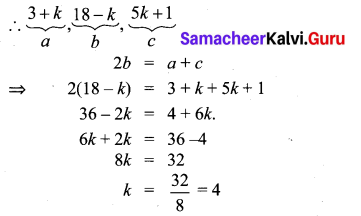10th Maths 2.5 Exercise Question 9.
Find x,y and z gave that the numbers x,
10, y, 24, z are in A.P.
x, 10, y, 24, z are in A.P
t2 – t1 = 10 – x
d = 10 – x …..(1)
t3 – t2 = y – 10
d = y – 10 ……(2)
t4 – t3 = 24 – y
d = 24 – y …..(3)
t5 – t4 = z – 24
d = z – 24 …..(4)
From (2) and (3) we get
y – 10 = 24 – y
2y = 24 + 10
2y = 34
y = 17
From (1) and (2) we get
10 – x = y – 10
– x – y = -10 -10
-x -y = -20
x + y = 20
x + 17 = 20(y = 17)
x = 20 – 17 = 3
From (1) and (4) we get
z – 24 = 10 – x
z – 24 = 10 – 3 (x = 3)
z – 24 = 7
z = 7 + 24
z = 31
The value of x = 3, y = 17 and z = 31

10th Maths 2.5 Question 10.
In a theatre, there are 20 seats in the front row and 30 rows were allotted. Each successive row contains two additional seats than its front row. How many seats are there in the last row?
Solution:
t1 = a = 20
t2 = a + 2 = 22
t3 = a + 2 + 2 = 24 ⇒ d = 2
∴ There are 30 rows.
t30 = a + 29d
= 20 + 29 × 2
= 20 + 58
= 78
∴ There will be 78 seats in the last row.

Exercise 2.5 Class 10 Question 11.
The Sum of three consecutive terms that are in A.P. is 27 and their product is 288. Find the three terms.
Let the three consecutive terms be a – d, a and a + d
By the given first condition
a – d + a + a + d = 27
3a = 27
a = $$\frac { 27 }{ 3 }$$ = 9
Again by the second condition
(a – d) (a) (a + d) = 288
a (a2 – d2) = 288
9(81 – d2) = 288 (a = 9)
81 – d2 = $$\frac { 288 }{ 9 }$$
81 – d2 = 32
∴ d2 = 81 – 32
= 49
d = $$\sqrt { 49 }$$ = ± 7
When a = 9, d = 7
a + d = 9 + 7 = 16
a = 9
a – d = 9 – 7 = 2
When a = 9, d = -7
a + d = 9 – 7 = 2
a = 9
a – d = 9 – (-7) = 9 + 7 = 16
The three terms are 2, 9, 16 (or) 16, 9, 2

Maths Exercise 2.5 Class 10 Question 12.
The ratio of 6th and 8th term of an A.P is 7:9. Find the ratio of 9th term to 13th term.
Solution:
$$\frac{t_{6}}{t_{8}}=\frac{7}{9}$$
$$\frac { a+5d }{ a+7d }$$ = $$\frac { 7 }{ 9 }$$
9a + 45d = 7a + 49d
9 a + 45 – 7d = 7a + 49 d
9a + 45d – 7a – 49d = 0
2a – 4d = 0 ⇒ 2a = 4d
a = 2d
Substitue a = 2d in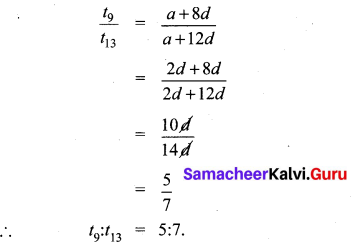Exercise 2.5 Class 10 Maths Samacheer Question 13.
In a winter season let us take the temperature of Ooty from Monday to Friday to be in A.P. The sum of temperatures from Monday to Wednesday is 0° C and the sum of the temperatures from Wednesday to Friday is 18° C. Find the temperature on each of the five days.
Solution:
Let the five days temperature be (a – d), a, a + d, a + 2d, a + 3d.
The three days sum = a – d + a + a + d = 0
⇒ 3a = 0 ⇒ a = 0. (given)
a + d + a + 2d + a + 3d = 18
3a + 6d = 18
3(0) + 6 d = 18
6d = 18
d = $$\frac { 18 }{ 6 }$$ = 3
∴ The temperature of each five days is a – d, a, a + d, a + 2d, a + 3d
0 – 3, 0, 0 + 3, 0 + 2(3), 0 + 3(3) = -3°C, 0°C, 3°C, 6°C, 9°C

2.5 Exercise Class 10 Question 14.
Priya earned ₹ 15,000 in the first month. Thereafter her salary increased by ₹1500 per year. Her expenses are ₹13,000 during the first year and the expenses increases by ₹900 per year. How long will it take for her to save ₹20,000 per month.
Solution: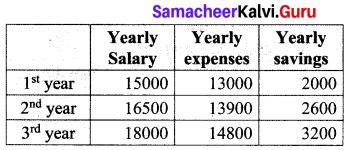We find that the yearly savings is in A.P with a1 = 2000 and d = 600.
We are required to find how many years are required to save 20,000 a year …………..
an = 20,000
an = a + (n – 1)d
20000 = 2000 + (n – 1)600
(n – 1)600 = 18000
n – 1 = $$\frac { 18000 }{ 600 }$$ = 30
n = 31 years